# Machine Learning 101 – Multiple Linear Regression

You already know of Simple Linear Regression. You also know of Logistic Regression. Now we would discuss Multiple Linear Regression.

This is a case where we have more than one predictor(independent) variables.

Let’s illustrate using the advertising dataset. You can get this dataset from this link. I’ts a csv file.  Just download it, so you can use it for this lesson.

A cross-section of the dataset is shown below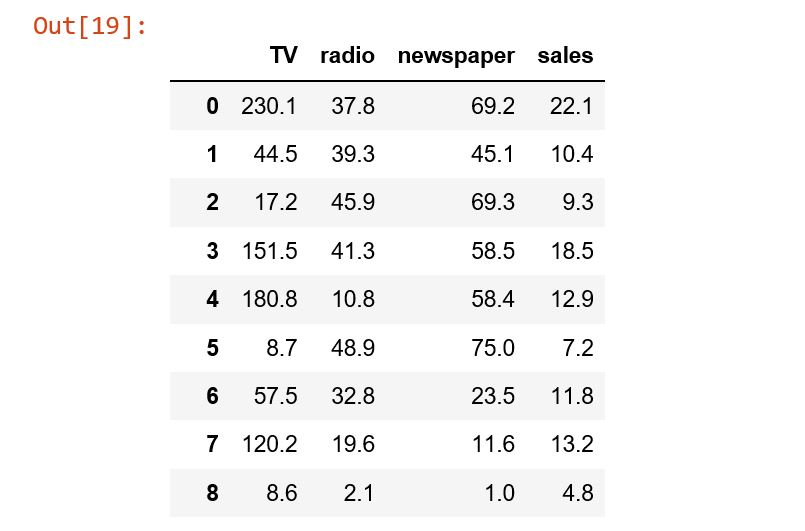Meanwhile to view the dataset: first download the advertising dataset to your computer(I saved mine in D:/data/Advertising.csv).

Then use the code below to load it into Jupyter Notebook.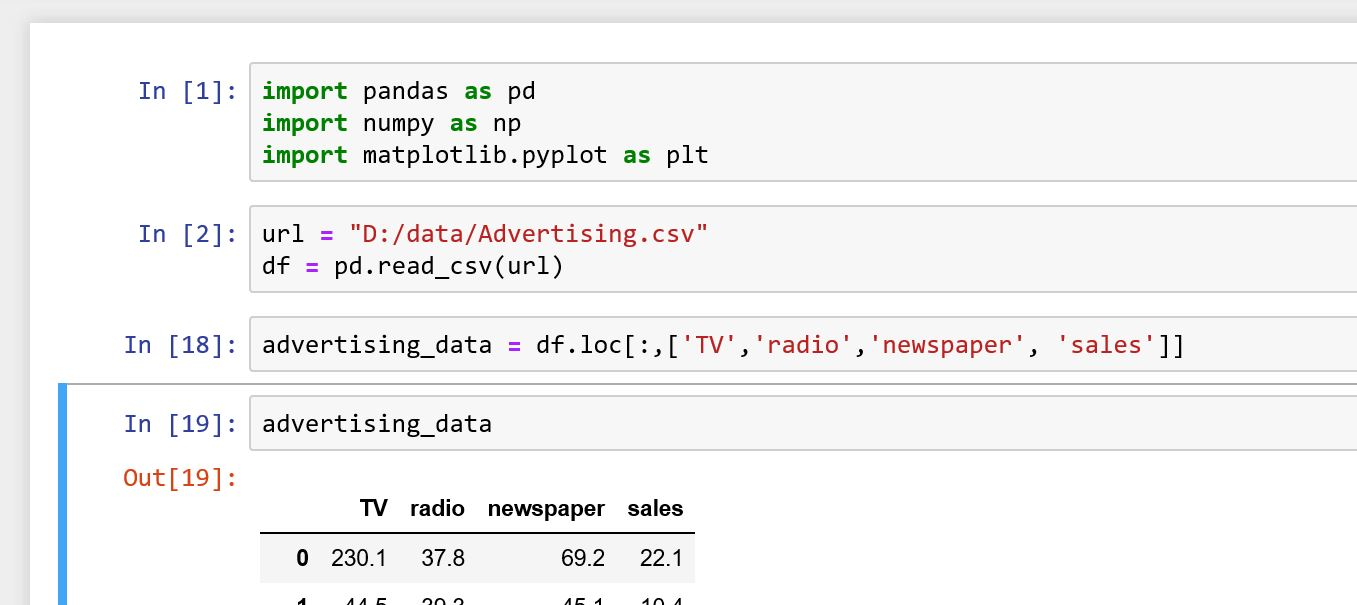In this dataset, we can see that there are three predictor variables: TV, radio and newspaper. Then we have one response variable: sales. We are interested in knowing how  the three predictor variables affect the response variable.

One way to do this is by running three separate linear regression lines, one for each of the 3 predictor variables.

So we can find how TV affects sales. In the case of TV we have

sales = 703.3 + 4.7TV

From the equation, we see that \$1,000 increase in spending on TV ads would result in \$4.57 units increase in sales.

Similarly, for radio and newspaper, we have:

sales = 931.2 + 202.5radio

sales = 1235.1 + 5.47newspaper

You can watch the video lesson to see how these is obtained.

The problem with Linear Regression

The challenge with this approach is that each of these equations ignores the effect of the other variables. Also, we don’t know how each of the variables affect the other. So in real scenario, we want to see how the three variables together affect sales. We can achieve this using multiple linear regression.

How Multiple Linear Regression Works

Multiple linear regression is an extension of linear regression. It simply has to now be adjusted to include multiple  predictor variables. Hence, in multiple linear regression, each predictor variable has its own coefficient all in a single model.

Assuming we have p predictor variables, then the multiple regression model will take the form:

Y = β0 + β1X1 +  β2X2 + . . . + βpXp  + 𝜖

Here,  Xj represents the jth predictor variable and βj indicates the relationship between that variable and the response.

Also, βj is interpreted as the average effect a unit increase in Xj will have on Y, while holding all other predictor variables fixed.

Now we can rewrite this model using TV, radio and newspaper as the predictor variables. So we have:

sales = β0 + β1 × TV + β2 × radio + β3 × newspaper + 𝜖

Now the question is:

How do we determine the regression coefficients for multiple regression? We would examine this in the next Lecture.

#### Python Practicals

In this practical, we would import the advertising data to Jupyter notebook.

Next, we would do a subplot of:

• TV vs sales
• radio vs sales
• newspaper vs sales

Then we would perform separate linear regression for each of them.

Finally we fit a regression line through each of the plots and determine the effect each of the predictor variables have on the value of sales.

You can find the screenshot of this practical below.

1. Import dataset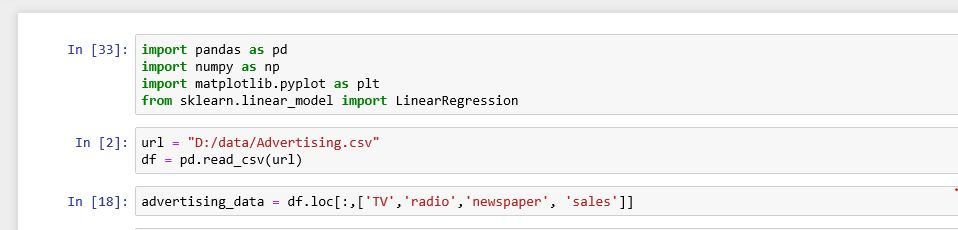2. Do a scatter plot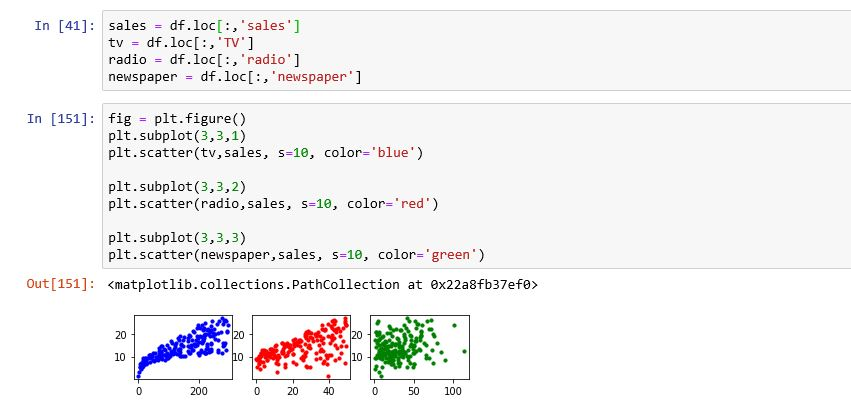3. Perform separate linear regression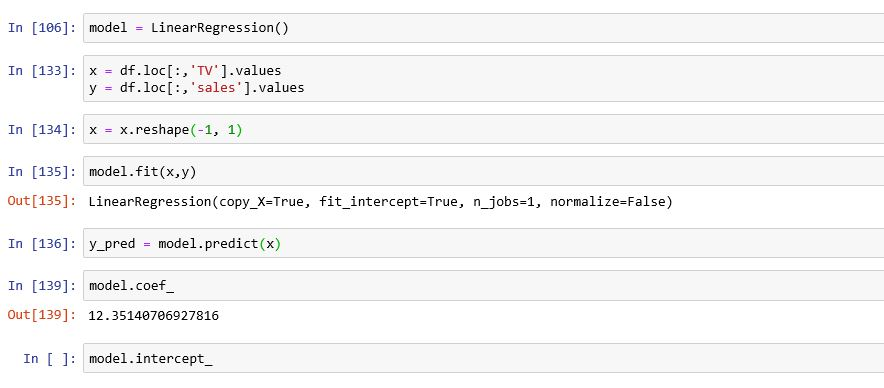#### kindsonthegenius

Kindson Munonye is currently completing his doctoral program in Software Engineering in Budapest University of Technology and Economics

View all posts by kindsonthegenius →